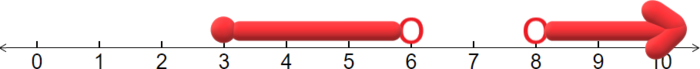$$\newcommand{\id}{\mathrm{id}}$$ $$\newcommand{\Span}{\mathrm{span}}$$ $$\newcommand{\kernel}{\mathrm{null}\,}$$ $$\newcommand{\range}{\mathrm{range}\,}$$ $$\newcommand{\RealPart}{\mathrm{Re}}$$ $$\newcommand{\ImaginaryPart}{\mathrm{Im}}$$ $$\newcommand{\Argument}{\mathrm{Arg}}$$ $$\newcommand{\norm}{\| #1 \|}$$ $$\newcommand{\inner}{\langle #1, #2 \rangle}$$ $$\newcommand{\Span}{\mathrm{span}}$$

# 6.3: The Union and Intersection of Two Sets

$$\newcommand{\vecs}{\overset { \rightharpoonup} {\mathbf{#1}} }$$ $$\newcommand{\vecd}{\overset{-\!-\!\rightharpoonup}{\vphantom{a}\smash {#1}}}$$$$\newcommand{\id}{\mathrm{id}}$$ $$\newcommand{\Span}{\mathrm{span}}$$ $$\newcommand{\kernel}{\mathrm{null}\,}$$ $$\newcommand{\range}{\mathrm{range}\,}$$ $$\newcommand{\RealPart}{\mathrm{Re}}$$ $$\newcommand{\ImaginaryPart}{\mathrm{Im}}$$ $$\newcommand{\Argument}{\mathrm{Arg}}$$ $$\newcommand{\norm}{\| #1 \|}$$ $$\newcommand{\inner}{\langle #1, #2 \rangle}$$ $$\newcommand{\Span}{\mathrm{span}}$$ $$\newcommand{\id}{\mathrm{id}}$$ $$\newcommand{\Span}{\mathrm{span}}$$ $$\newcommand{\kernel}{\mathrm{null}\,}$$ $$\newcommand{\range}{\mathrm{range}\,}$$ $$\newcommand{\RealPart}{\mathrm{Re}}$$ $$\newcommand{\ImaginaryPart}{\mathrm{Im}}$$ $$\newcommand{\Argument}{\mathrm{Arg}}$$ $$\newcommand{\norm}{\| #1 \|}$$ $$\newcommand{\inner}{\langle #1, #2 \rangle}$$ $$\newcommand{\Span}{\mathrm{span}}$$

Learning Outcomes

1. Find the union of two sets.
2. Find the intersection of two sets.
3. Combine unions intersections and complements.

All statistics classes include questions about probabilities involving the union and intersections of sets. In English, we use the words "Or", and "And" to describe these concepts. For example, "Find the probability that a student is taking a mathematics class or a science class." That is expressing the union of the two sets in words. "What is the probability that a nurse has a bachelor's degree and more than five years of experience working in a hospital." That is expressing the intersection of two sets. In this section we will learn how to decipher these types of sentences and will learn about the meaning of unions and intersections.

## Unions

An element is in the union of two sets if it is in the first set, the second set, or both. The symbol we use for the union is $$\cup$$. The word that you will often see that indicates a union is "or".

Example $$\PageIndex{1}$$: Union of Two sets

Let:

$A=\left\{2,5,7,8\right\} \nonumber$

and

$B=\lbrace1,4,5,7,9\rbrace \nonumber$

Find $$A\cup B$$

Solution

We include in the union every number that is in A or is in B:

$A\cup B=\left\{1,2,4,5,7,8,9\right\} \nonumber$

Example $$\PageIndex{2}$$: Union of Two sets

Consider the following sentence, "Find the probability that a household has fewer than 6 windows or has a dozen windows." Write this in set notation as the union of two sets and then write out this union.

Solution

First, let A be the set of the number of windows that represents "fewer than 6 windows". This set includes all the numbers from 0 through 5:

$A=\left\{0,1,2,3,4,5\right\} \nonumber$

Next, let B be the set of the number of windows that represents "has a dozen windows". This is just the set that contains the single number 12:

$B=\left\{12\right\} \nonumber$

We can now find the union of these two sets:

$A\cup B=\left\{0,1,2,3,4,5,12\right\} \nonumber$

## Intersections

An element is in the intersection of two sets if it is in the first set and it is in the second set. The symbol we use for the intersection is $$\cap$$. The word that you will often see that indicates an intersection is "and".

Example $$\PageIndex{3}$$: Intersection of Two sets

Let:

$A=\left\{3,4,5,8,9,10,11,12\right\} \nonumber$

and

$B=\lbrace5,6,7,8,9\rbrace \nonumber$

Find $$A\cap B$$.

Solution

We only include in the intersection that numbers that are in both A and B:

$A\cap B=\left\{5,8,9\right\} \nonumber$

Example $$\PageIndex{4}$$: Intersection of Two sets

Consider the following sentence, "Find the probability that the number of units that a student is taking is more than 12 units and less than 18 units." Assuming that students only take a whole number of units, write this in set notation as the intersection of two sets and then write out this intersection.

Solution

First, let A be the set of numbers of units that represents "more than 12 units". This set includes all the numbers starting at 13 and continuing forever:

$A=\left\{13,\:14,\:15,\:...\right\} \nonumber$

Next, let B be the set of the number of units that represents "less than 18 units". This is the set that contains the numbers from 1 through 17:

$B=\left\{1,\:2,\:3,\:...,\:17\right\} \nonumber$

We can now find the intersection of these two sets:

$A\cap B=\left\{13,\:14,\:15,\:16,\:17\right\} \nonumber$

## Combining Unions, Intersections, and Complements

One of the biggest challenges in statistics is deciphering a sentence and turning it into symbols. This can be particularly difficult when there is a sentence that does not have the words "union", "intersection", or "complement", but it does implicitly refer to these words. The best way to become proficient in this skill is to practice, practice, and practice more.

Example $$\PageIndex{5}$$

Consider the following sentence, "If you roll a six sided die, find the probability that it is not even and it is not a 3." Write this in set notation.

Solution

First, let A be the set of even numbers and B be the set that contains just 3. We can write:

$A=\left\{2,4,6\right\},\:\:\:B\:=\:\left\{3\right\} \nonumber$

Next, since we want "not even" we need to consider the complement of A:

$A^c=\left\{1,3,5\right\} \nonumber$

Similarly since we want "not a 3", we need to consider the complement of B:

$B^c=\left\{1,2,4,5,6\right\} \nonumber$

Finally, we notice the key word "and". Thus, we are asked to find:

$A^c\cap B^c=\:\left\{1,3,5\right\}\cap\left\{1,2,4,5,6\right\}=\left\{1,5\right\} \nonumber$

Example $$\PageIndex{6}$$

Consider the following sentence, "If you randomly select a person, find the probability that the person is older than 8 or is both younger than 6 and is not younger than 3." Write this in set notation.

Solution

First, let A be the set of people older than 8, B be the set of people younger than 6, and C be the set of people younger than 3. We can write:

$A=\left\{x\mid x>8\right\},\:\:\:B\:=\:\left\{x\mid x<6\right\},\:C=\left\{x\mid x<3\right\} \nonumber$

$A\cup\left(B\cap C^c\right) \nonumber$

Notice that the complement of "$$<$$" is "$$\ge$$". Thus:

$C^c=\left\{x\mid x\ge3\right\} \nonumber$

Next we find:

$B\cap C^c=\left\{x\mid x<6\right\}\cap\left\{x\mid x\ge3\right\}=\left\{x\mid3\le x<6\right\} \nonumber$

Finally, we find:

$A\cup\left(B\cap C^c\right)=\:\left\{x\mid x>8\right\}\cup\left\{x\mid3\le x<6\right\} \nonumber$

The clearest way to display this union is on a number line. The number line below displays the answer:Exercise

Suppose that we pick a person at random and are interested in finding the probability that the person's birth month came after July and did not come after September. Write this event using set notation.

6.3: The Union and Intersection of Two Sets is shared under a CC BY-NC-SA license and was authored, remixed, and/or curated by Larry Green.